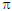# Electronic Devices - BJT and FET Frequency Response

### Exercise :: BJT and FET Frequency Response - General Questions

26.

logea = ________ log10a

 A. 2.3 B. 2.718 C. e D. 1.414

Explanation:

No answer description available for this question. Let us discuss.

27.

In the hybridor Giacoletto model, which one of the following does rb include?

 A. Base spreading resistance B. Base contact C. Base bulk D. All of the above

Explanation:

No answer description available for this question. Let us discuss.

28.

What is the ratio of the output voltage to the input voltage at the cutoff frequencies in a normalized frequency response plot?

 A. 0.25 B. 0.5 C. 0.707 D. 1

Explanation:

No answer description available for this question. Let us discuss.

29.

Which of the following statements is true for a square-wave signal?

 A. It is composed of both even and odd harmonics. B. It is composed only of odd harmonics. C. It is composed only of even harmonics. D. The harmonics waveforms are also square waves.

Explanation:

No answer description available for this question. Let us discuss.

30.

A change in frequency by a factor of ________ is equivalent to 1 decade.

 A. 2 B. 10 C. 5 D. 20